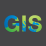# Field calculator if statement syntax error.

769
3
07-23-2021 03:28 PMNew Contributor II

I'm using ArcGIS Pro 2.7.

I'm trying to use a python function in the field calculator; which I've done before.  I've got a field with data type Double that we'll call Double_Field that I want to use to determine which value to assign to another field.  Here's what I'm trying:

NewValue(!Double_Field!)

def NewValue(num):
if num = 0:
return 1
elif num > 0 and num <= 3:
return 2
elif num > 3 and num <= 6:
return 3
elif num > 6 and num <= 9:
return 4
elif num > 9 and num <= 12:
return 5
elif num > 12:
return 6
else:
return 99

When I try to verify the code I get the following errorI've noticed that if I change the "if num = 0:" to "if num < 0:" or anything but a "=" comparison I no longer get a syntax error (but then code no longer does what I need it to do).

Can anyone help me understand what's going on here?  I'm a little new to Python but not to programming.  It feels a little like a type error but I cannot see how.

Thanks,

Michael Marlatt

1 Solution

Accepted SolutionsOccasional Contributor III

In Python the equals comparison is written as ==, so use

``if num == 0:``

3 RepliesOccasional Contributor III

In Python the equals comparison is written as ==, so use

``if num == 0:``New Contributor II

Thank you, Tim!byMVP Esteemed Contributor

Another case for divmod

``````def NewValue(num, divisor=3):
"""Return divmod for a field"""
val = divmod(num, divisor)
if val > 6:
return 99
return val

[NewValue(i) for i in a]
[0, 1, 2, 3, 4, 5, 6, 99, 99, 99]

a
array([ 0,  3,  6,  9, 12, 15, 18, 21, 24, 27])``````

... sort of retired...